﻿ Triplet of alkaline-earth metals (Mg,Ca,Sr,Ba)

# Spin "triplet" includes NO "Spin".

## Triplet, Singlet of metals have Nothing to do with "Spin".

### [ Spin-spin (- orbit ) interactions are too weak to explain "triplet" and "singlet". ]

(Fig.1) Triplet, Singlet of Magnesium (= Mg ) mean large "Coulomb" energy ( NOT spin ).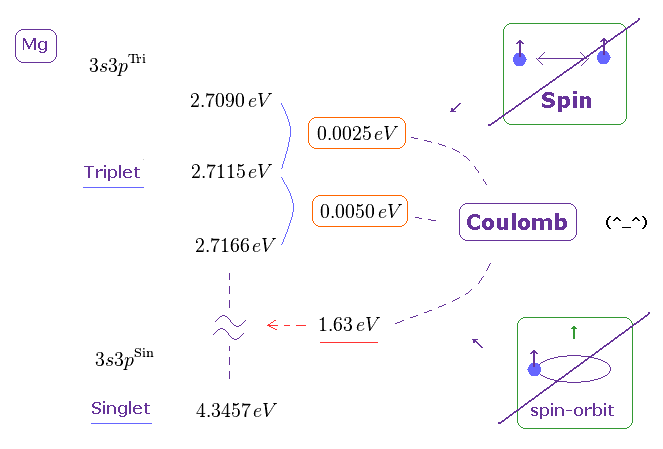The current quantum theory insists that "triplet" and "singlet" states of the alkaline earths are caused by spin-spin and spin-orbit interactions.
But in fact, the energy intervals in singlet and triplet are much bigger than the magnetic energies among spins.
( The Story of Spin by S.Tomonaga says they are as big as those of electric origin, as shown on this page. )

On this page, we calculate the magnitude of the energies based on spin-spin and spin-orbit interactions, and compare them with the experimental values.
After that, you will find that the concept of "Spin" in triplet and singlet is just an illusion.

## What is "triplet" and "singlet" states ?

(Fig.2) One of two valence electrons is excited to 3p level in Mg.Basically, in singlet and triplet states, only one of two valence electrons of magnesium is excited to upper energy levels such as 3p, 3d, 4s .., as shown in Nist.
( Another valence electron remains in the lowest 3s level. )

In this state, when these two spins turn in the same directions, it is called "triplet".
And when these two spins are antiparallel to each other, it's called "singlet".
But in fact, the "spin" has nothing to do with these states, as I explain in this page.

(Fig.3) Two spins are parallel. → S = 1/2 + 1/2 = 1.Each spin has 1/2 angular momentum.
So in triplet state, total spin become S = 1/2 + 1/2 = 1, because they are parallel to each other.
Only 3p orbtial has orbital angular momentum ( L = 1 ).

Depending on whether total spin is parallel or antiparallel to the 3p orbital motion (= L ), it's split into three energy levels, they insist.

## Triplet, Singlet are just "Math" symbols with NO physical images.

(Fig.4) Triplet state ?   ← Only "Math", NOT physics.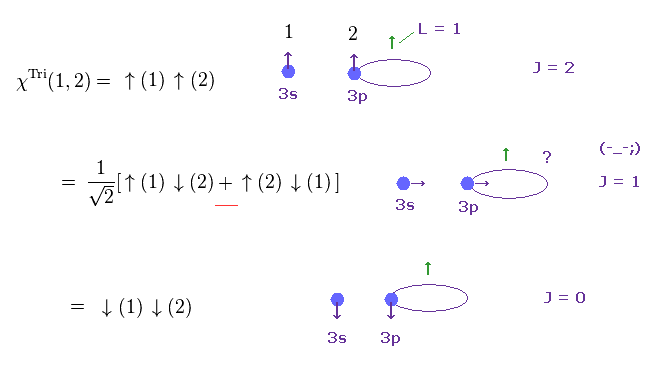In Fig.4 upper, two spins ( S = 1 ) are parallel to the orbital angular momentum ( L = 1 ).
As a result, total angular momentum becomes J = S + L = 2.
In the lowest figure, two spins are antiparallel to the orbital, so its total J = L - S = 0.

The problem is the intermediate state (= middle of Fig.4 ).
Of course, if we admit transverse direction of spin, it means all arbitrary directions are allowed, and it can be split into infinite states.

In the intermediate state, one spin is "up", and another spin is "down".
And the spin wavefunction (= χ ) of Fig.4 is symmetric, as shown on this site.
(= Even if you exchange the numbers of "1" and "2" in Fig.4, the sign remains the same. )
But this is just "mathematical" reason without physical images at all.

(Fig.5) Singlet state ?   ← What is the physical meaning of ± sign ?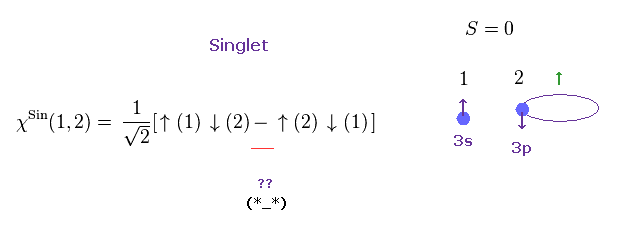As I said in Fig.4, in the intermediate state of triplet, one electron is "up", and another is "down".
Surprisingly, also in the singlet state, one electron is "up", and another is "down".
Then what is the difference between triplet and singlet ?

In the singlet state, the spin wavefunction (= χ ) is antisymmetric.
When you exchange the numbers of "1" and "2" of this singlet χ, the total sign becomes the opposite.

## One is "up", another is "down" spin, in Both triplet and singlet ?

(Fig.6) In both triplet and singlet, one spin is "up", another spin is "down". ← What's the difference ?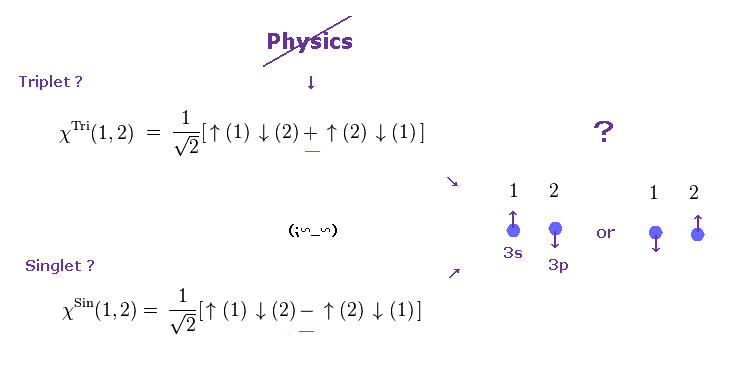But as you notice, these reasons (= symmetric or not ) are only mathematical, and lacks physical images.
If one spin is "up", and another spin is "down" in both triplet (= intermediate ) and singlet states, it means there are NO difference between them, from the realistic viewpoint.

These concepts were introduced only for explaining experimental results (= only splitting number ).
In fact, they completely ignored the magnitude of energies in triplet and singlet.
Because the experimental energy intervals are much bigger than spin-spin dipole interactions.

## Triplet, singlet are split by "Coulomb" energy ( NOT spin ! )

### [ Triplet splitting is "too large" to be explained by spin-spin interactions. ]

(Fig.7) Energy levels in triplet and singlet of magnesium.Each energy levels of triplet and singlet states of magnesium is in Nist.
The energy unit of "cm-1" can be changed into "eV", as shown in Eq.1 ( or this site. )

(Eq.1) Conversion of energy units.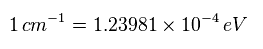As you see Fig.7, the energy difference between triplet and singlet is as big as 1.63 eV.
This value is much bigger than weak spin interaction ( 1.63 eV > 0.000045 eV in hydrogen. See this site. )
And in magnesium n = 3 orbitals, this energy becomes as small as 0.00002 eV.
So this energy interval clearly originates from Coulomb interaction ( NOT spin magnetic moment ! ).

## Triplet splitting is NOT by "spin".

(Fig.8) Spin-spin magnetic dipole energy is too small to explain triplet splitting.Also in triplet splitting in magnesium, the energy intervals are much larger than the spin-spin magnetic dipole interaction.
For example, when two electrons' spins are separated from each other by 1.0 × 10-10 meter (= about 2 × Bohr radius ), this spin-spin magnetic energy becomes 1.7 × 10-23 J (= 0.00010 eV ), as shown on this site (33.10).

(Fig.9) Spin-spin magnetic energy is too small.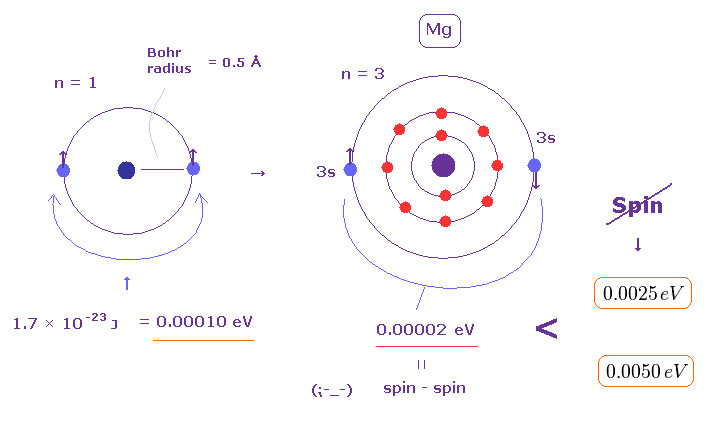Bohr radius is the average radius of hydrogen ground state ( n = 1 ).
Valence electron of magnesium is in n = 3 orbital.
So the distance between two spins in Mg outer orbitals is much longer than Bohr radius.

As you see Fig.9, these spin-spin magnetic energies (= 0.00002 eV ) cannot reach the exprimental triplet splitting (= 0.0050 eV ) at all.

## Spin-orbit interaction is also too weak to explain triplet.

(Fig.10) Spin-orbit interaction is too small to explain triplet splitting.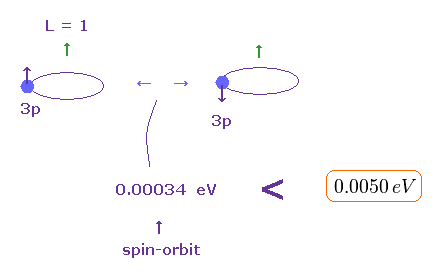As shown in Fig.4, the electron spin in 3p orbital becomes parallel or antiparallel to the orbital angular momentum.
So spin-orbit coupling energy may be involved in this triplet splitting.

But if you calculate this effect of spin-orbit interaction, you will easily find this effect is also too small to explain triplet splitting.
0.00034 eV (= spin-orbit ) vs. 0.00500 eV (= experimental value ).

## Triplet, singlet splitting are due to strong Coulomb force.

(Fig.11) Spin-orbit interaction is too small to explain triplet and singlet splitting.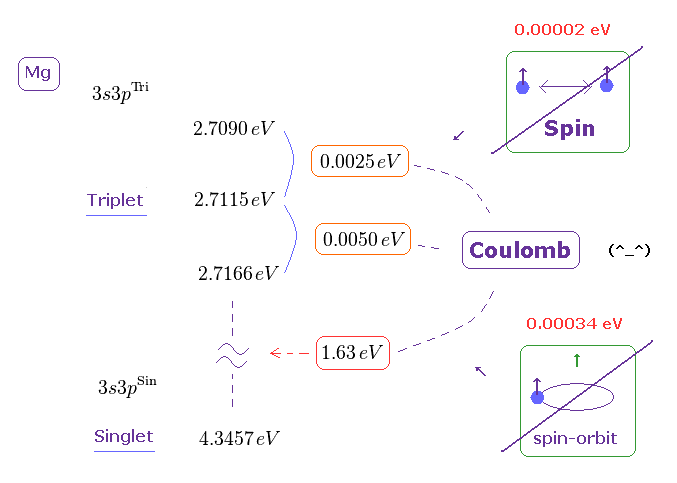As a result, we find that these triplet and singlet energy splitting are caused by strong Coulomb interactions, neither by spin-spin nor spin-orbit coupling.

In spite of these obvious facts, usual textbooks and schools tend to hide this truth from ordinary people and students.
This is very dangerous, and a kind of frauds, I think.

## Spin-spin magnetic dipole energy cannot explain Pauli exclusion principle and Triplet.

### [ Spin-spin dipole magnetic energies ( < 0.0001 eV ) are far weaker than strong Pauli repulsive energies ( > 30 eV) and ferromagnet ( > 1 eV ). ]

(Fig.12) Spin-spin magnetic dipole energy is too small ( < 0.0001 eV )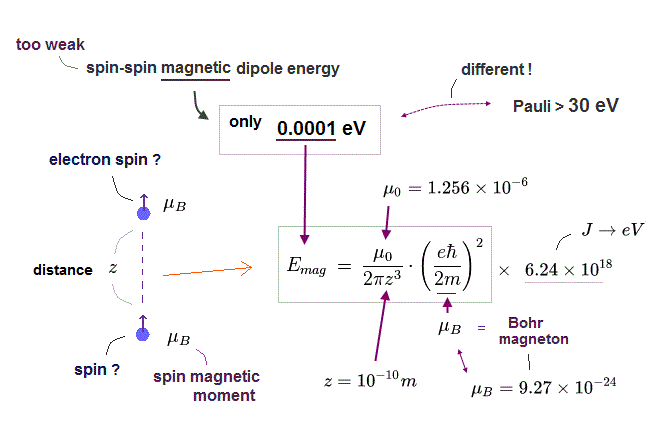Here we calculate spin-spin magnetic dipole energy or interaction and show the spin magnetic interaction is too weak (= spin magnetic energy is too small ) to explain strong Pauli exclusion repulsion and ferromagnetism, so electron spin is unreal.

When the distance between two electron spins (= spin magnet = Bohr magneton ) is "z ( or distance r apart )", its magnetic energy (= Emg ) becomes like Fig.12. See also this,  this p.17,  this, this-lower . Each spin magnetic moment is Bohr magneton (= μB )

Unrealistic electron spin is accidentally supposed to have the same magnetic moment = Bohr magneton as the Bohr's atomic orbit, as shown in this-lower.

Using these fundamental constants, you can calculate and estimate spin-spin magnetic dipole energy, which is far smaller (= about 0.0001 eV, when the distance between two spin magnetic moments is about 1 Å = 1 × 10-10 meter ) than energies required to generate strong Pauli exclusion repulsion (= about 30 eV ) or ferromagnetism (= 1 eV ), so electron spin is unreal.

(Fig.13) Spin-spin magnetic dipole energy. Part II.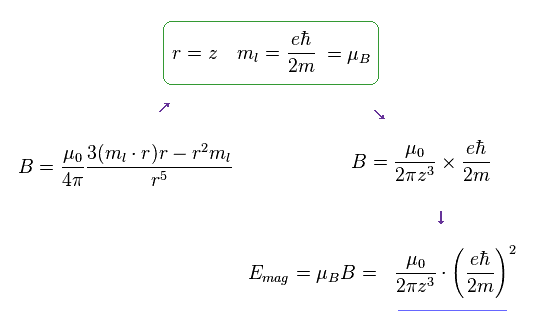When the direction of "z" is different from spin, the generalized form of magnetic field (= B ) caused by spin becomes like Fig.13.
When "r" is (anti)parallel to ml (= magnetic moment ), this form is just equal to Fig.12.
So we use simple Fig.12 as spin-spin magnetic energy.

### [ Magnetic field (= B ) generated by circular current I. ]

(Fig.14)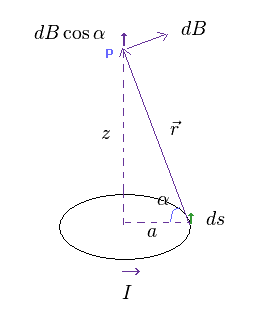In Fig.14, the circular current I generates the magnetic field (= B ) at the point of "p".
Due to this circular elecric orbit, all magnetic fields other than z direction are cancelled out.
Only the z conponent (= dB cos α ) is left.

(Eq.2)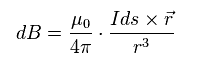According to Biot-Savart law ( see this site ), magnetic field (= B ) is as shown in Eq.2.
"I" is electric current, "ds" is electric current fragment, and "r" is the distance between this fragment and the point "p".

(Eq.3)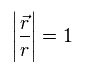In Eq.2, the unit vector in "r" direction is Eq.3.
Using Eq.3 in Eq.2, the z component of total magnetic field becomes

(Eq.4)where
(Eq.5)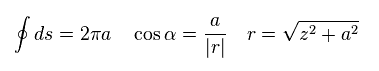The result of Eq.4 is the same as this site.
Using Eq.5 and the definition of Iπa2 = magnetic moment (= Bohr magneton ), Eq.4 becomes Eq.6.
( About elliptical orbit, see this section. )

(Eq.6)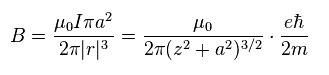The magnetic energy (= Emg ) is given by magnetic moment (= μB ) × B.
Considering this μB is Bohr magneton, this energy becomes

(Eq.7)   Spin-Spin magnetic energy.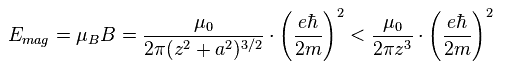If the circular current is spin, the orbital radius "a" is almost zero in Eq.7 right ( a → 0 ).
( Of course, when this "a" is not zero, this magnetic energy becomes smaller, as shown in Eq.7. )
Eq.7 result is the same as this site (33.9).

If we can know the distance (= z ) between two spins in outer orbitals of magnesium, the magnetic energy of Eq.7 is determined.

### [ Calculation of the estimated "3s" radius (= r ) of Magnesium. ]

(Fig.15) Estimated radius of 3s orbital in Mg.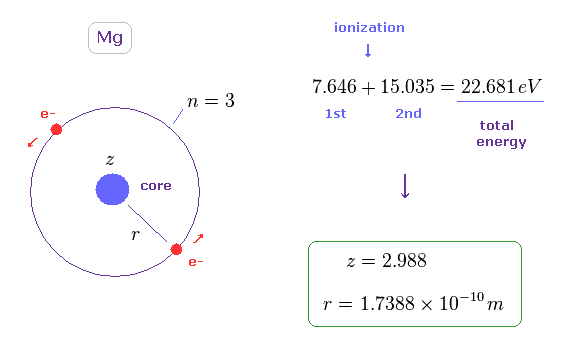To know the average distance between two spins in outer orbitals of Mg, we use the simple model of Fig.15.
In Fig.15, two ( outer ) electrons are on the opposite sides of the core (= effective positive charge "z" ), and moving on the same orbit (= 3 × de Broglie wavelength ).

This simple model can give the results close to experimental values, as shown on this page (Table 1).
We use the sum of the 1st and 2nd ionization energies ( 7.646 + 15.035 = 22.681 eV, see this site ) as the total energy ( E = - 22.681 eV ).

Using this total energy, we obtain the radius of r = 1.7388 Å, and the central effective charge z = 2.988.
This radius is almost same as this site.
About the detailed calculation, see this section.

### [ Rough estimation of radius. ]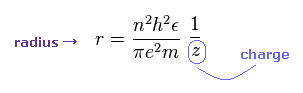The orbital radius is proportional to a square of principal number (= n2 ), and in inverse proportion to the central charge (= z ), as shown in this site (Eq.5).

(Fig.16) Rough estimation of radius of Mg 3s orbital ( z = 3 ).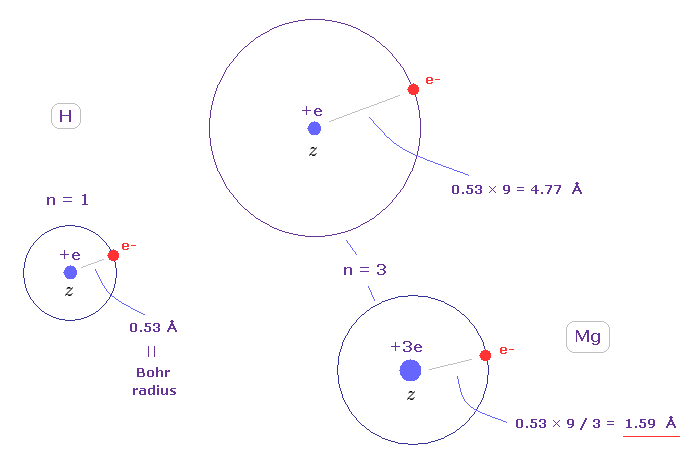The atomic radius of the ground state hydrogen ( z = 1, n = 1 ) is just Bohr radius (= 0.53 Å ).
So the orbital length of n = 3 is 9 × 0.53 = 4.77 Å.

Suppose the central charge of Mg2+ is about z = 3 ( this z becomes a little bigger than "2" due to "gap" of inner orbitals ).
This Mg radius becomes 0.53 × 9 / 3 = 1.59 Å, which is similar to the result of 1.738 Å of Fig.15.
( Considering the repulsive effect of another outer electron, the actual effective charge becomes less than "3". )

### [ Spin-spin magnetic dipole energy in Mg. ]

(Fig.17)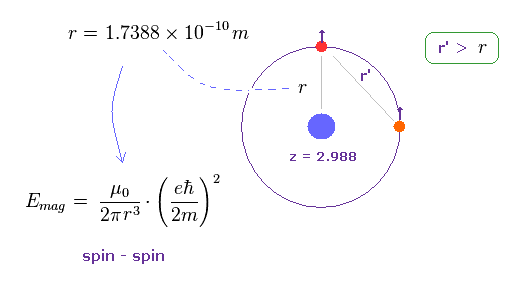"r" of Fig.15 is radius of outer orbital of Mg.
So the distance (= r' ) between two valence electrons is longer than this radius, as shown in Fig.17 ( r' > r ).

So if spin-spin magnetic energy based on this distance "r" is smaller than triplet splitting, this magnetic energy cannot reach the experimental values.

(Eq.8)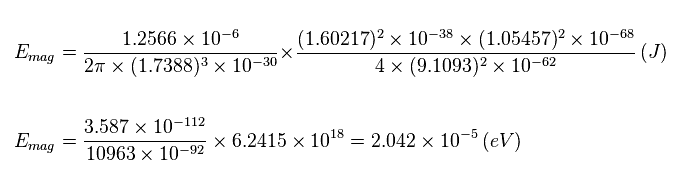So, the spin-spin magnetic dipole energy is less than 2.042 × 10-5 eV.
This value is very small, which is about 1/100 times the actual triplet splitting (= 0.0025 or 0.0050 eV, see Fig.7 ).

(Fig.18) Spin-Spin magnetic energy is too small.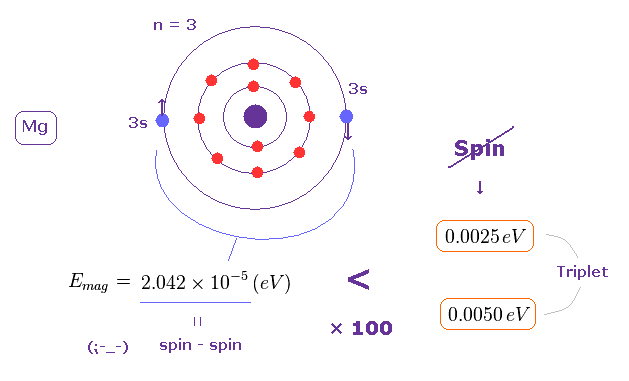You can easily understand it is impossible to explain triplet splitting using spin-spin magnetic interaction.
In spite of this fact, they never give up this strange "spin".
This is the reason why they keep troublesome "Shut up and calculate !" attitude about what spin really is.

## Spin-orbit coupling cannot explain Triplet.

(Fig.19) Spin-orbt coupling is too small, too.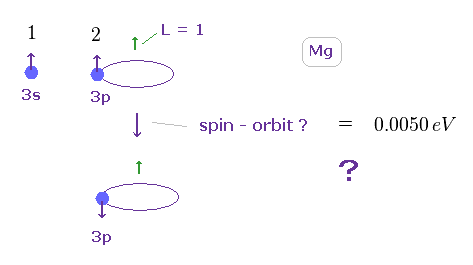In the above section, we explain spin-spin magnetic dipole energies are so small that they cannot explain actual triplet ( and singlet ) energy splitting.
Only 3p orbital has angular momentum ( L = 1 ) in 3s3p electron pair of Mg.
So the flipping over electron's spin in 3p orbital may cause spin-orbit coupling energy.

But when you calculate spin-orbit coupling energy in magnesium 3p orbital, you will find this coupling energy is too small to explain triplet ( singlet ) energy spilitting (= 0.0050 eV, see Fig.7 ).
So, " triplet ( or singlet ) = spin " proves to be just an illusion.

(Fig.20) Doublet of hydrogen (= H ) and triplet of magnesium (= Mg ).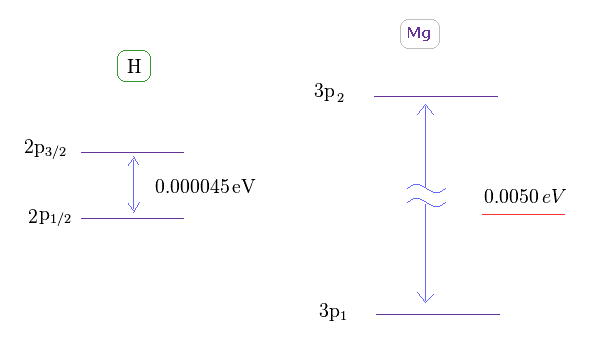As shown on this site ( or Nist ), hydrogen fine structure (= doublet ) between 2p3/2 and 2p1/2 is about 0.000045 eV.
On the other hand, triplet energy splitting of magnesium is as large as 0.0050 eV. See Nist.

According to the current quantum mechanics, triplet splitting must be caused by change of spin directions in 3s-3p orbitals.
But it turns out to be that this large interaval is NOT by very weak spin magnetic moment.

### [ Magnesium triplet is caused by spin-orbit coupling → Z becomes too big ( 2 → 4.40 ). ]

(Fig.21) Core charge of magnesium is much bigger then Z = 2 !?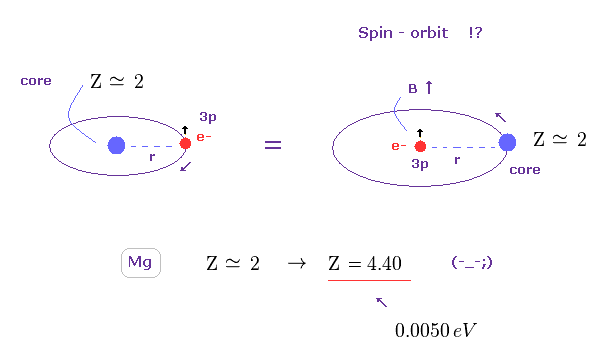The magnesium has two valence electrons ( in this case, one is 3s, and another is excited to 3p ).
So the Ma2+ ion has about Z = +2 central core charge.
From the viewpoint of 3p electron, core charge Z is moving around 3p electron, and generates magnetic field (= B ), which is the origin of spin-orbit magnetic energy, they insist.

But if you try to get triplet energy splitting (= 0.0050 eV ) using spin-orbit coupling, this central core charge becomes as big as Z = 4.40.
This result clearly shows spin-orbit coupling has nothing to do with Mg triplet ( of course, singlet ).

(Fig.22) Energy splitting (= ΔE ) by spin-orbit interaction.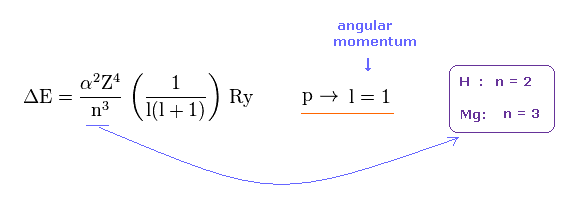It is known that energy splitting by spin-orbit coupling is proportional to Z4 ( Z is central charge ), and in inverse proportion to n3 ( n is principal quantum number ), as shown on this site (p.9) or this site .

Hydrogen doublet is n = 2 energy level. Magnesium in this case is n = 3 energy level.
Angular momentums of "p" orbital are the same in both atoms ( l = 1 in H and Mg ).

(Fig.23) How big is central core charge Z in Mg ?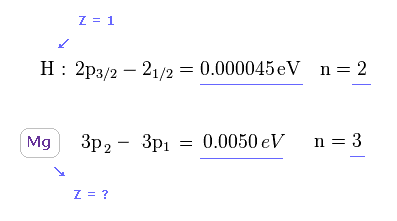As a result, the central core charge Z of Mg must be much bigger than H to explain large discrepancy between this energy splitting of Mg and H.
( Of course, central charge of H is Z = 1 ).

(Fig.24) Effective core charge of Mg becomes as large as Z = 4.40 !?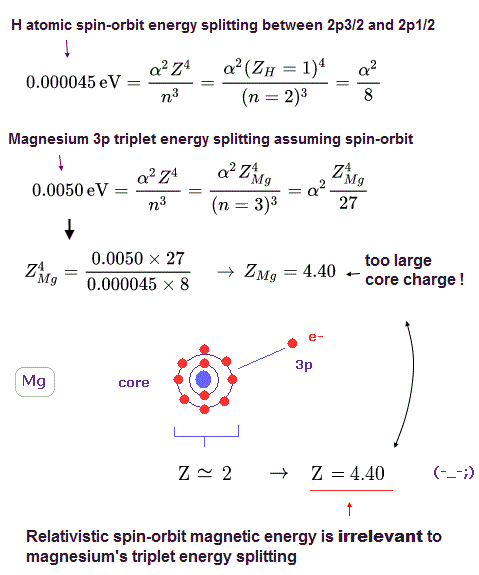Using Fig.22 and Fig.23 ( comparing the values of H and Mg ), we can know the central core charge Z of Mg.
As shown in Fig.24, this charge of Mg becomes as large as Z = 4.40 !

Of course, this central core charge Z = 4.40 is too big and unrealistic.
This calculation result clearly proves spin-orbit coupling has nothing to do with Mg triplet enegy splitting, because it's too weak.

### [ True central core charge by ionization energy. ]

(Fig.25) What is effective core charge Z in Mg ?We can know true effective core charge Z based on ionization energy of Mg.
The first ionization energy of Mg is 7.646 eV.
( This electron is "3s". Of course, "3p" electron is farther away from nucleus, so Z becomes smaller. )

(Fig.26)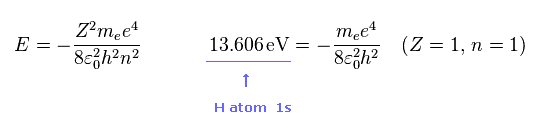As you know, hydrogen ionization energy of 1s electron is 13.606 eV.
In 1s hydrogen, Z = 1 and n = 1.

(Fig.27) What is effective core charge Z in Mg ?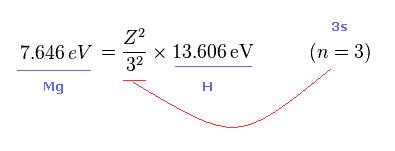From Fig.25 and Fig.26 we get the relation of Fig.27.
Z of Fig.27 means effective core charge from the viewpoint of Mg 3s electron.
( Caution: this Z contains Mg nucleus and all other electrons including another 3s electron. )

(Fig.28) Effective core charge Z in Mg.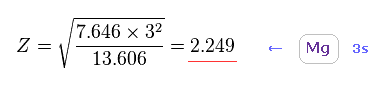Solving Fig.27, we get the true effective core charge Z = 2.249.
This Z also includes another 3s electron, so this is smaller than Fig.15.

(Fig.29) Wide discrepancy between true and "fake" core charges.Comparing Fig.28 ( true Z = 2.24 ) and Fig.24 ( Z = 4.40 by spin-orbit ), you can easily find that spin-orbit coupling is too weak to explain Mg triplet.

(Fig.30) Triplet, Singlet of Mg have Nothing to do with "Spin" .Using true effective core charge, spin-orbit coupling energy becomes as small as 0.00034 eV.
As you see Fig.30, the energy difference between singlet and triplet is much bigger (= 1.63 eV ).
So it is much more impossible to insist this wide energy gap is caused by very weak spin.

They insist this large enery gap (= 1.63 eV ) is due to the difference of symmetric and antisymmetric wavefunction, as shown in Fig.31 ( or see this site ).

(Fig.31) "Symmetric" and "antisymmetric" wavefunctions can cause large energy gap ?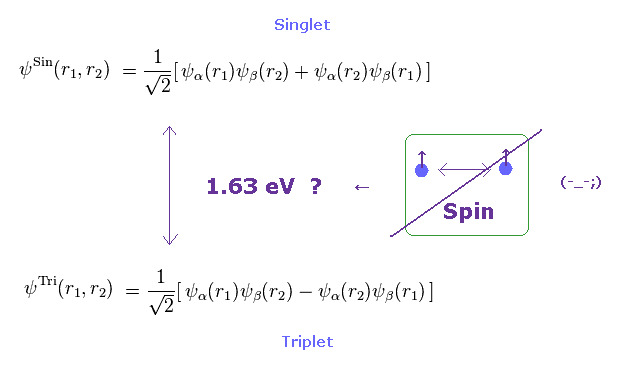But of course, as shown in the above sections, spin-spin interaction (= 0.00002 eV ) and spin-orbit coupling (= 0.00034 eV using true effective Z ) can NEVER reach as much as 1.63 eV.

In spite of this fact, the current physicists try to link singlet and triplet states to unrealistic "spin".
This misuse of "spin" invites misunderstanding among ordinary people, I'm afraid.

Inside triplet states, their wavefunctions are the common form (= antisymemtric, as shown in Fig.31 lower ).
So the triplet energy splitting cannot use this logic such as symmetric or antisymmetric.
The triplet must be explained purely by spin-spin ( or -orbit ) coupling, but they are too weak.
In conclusion, the magnesium triplet and singlet splitting are caused by strong Coulomb force ( NOT spin ).

## Calcium (= Ca ) triplet does NOT represent "Spin", either.

(Fig.32) Triplet, Singlet splitting in Ca is too large for spin-spin (-orbit) coupling.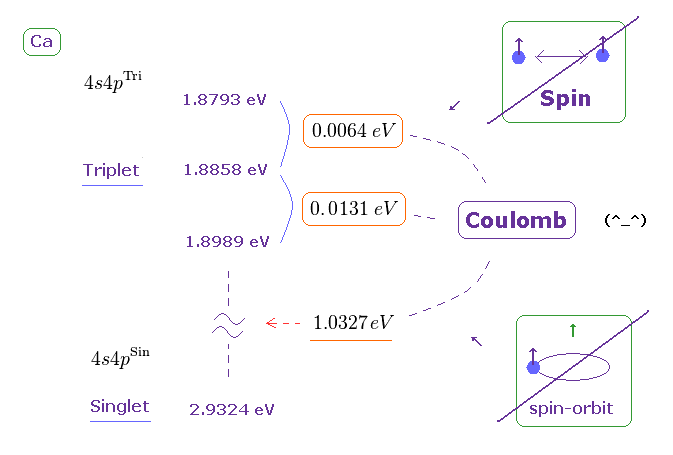Triplet and singlet energy levels are on this site.
Converting energy unit ( cm-1 → eV ) by
(Eq.9)We obtain Fig.32.
If you compare Fig.32 (= Ca ) with Fig.30 (= Mg ), you will understand energy splitting (in triplet ) in Ca is larger than Mg.
So it is much more impossible that spin-spin ( or -orbit ) coupling is involved in this triplet.

(Fig.33) Radius of helium-like model in Ca.The sum of 1st and 2nd ionization energy is 17.984 eV in Ca, as shown on this site.
Like Fig.15, we suppose helium-like model in Ca, and its total energy is -17.984 eV.

In this model, the radius (= r ) becomes 2.6036 Å, which is longer than Mg.
The calculation method is the same as this section.

(Fig.34) Spin-spin magnetic energy is 1/1000 times triplet splitting in Ca.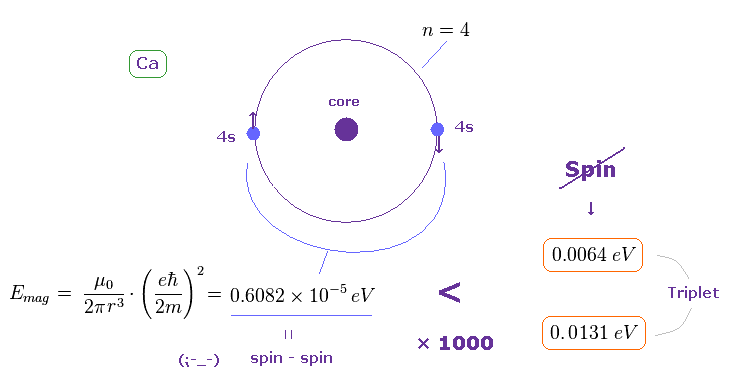Using the approximate radius of Fig.33, we find the spin-spin magnetic dipole energy in Ca outer orbital becomes very small.
(= 0.6082 × 10-5 eV, about the method, see this section. )
This result shows it is impossible to explain triplet splitting by spin-spin magnetic interactions also in calcium.

### [ How about spin-orbit coupling energy in Ca ? ]

(Fig.35) Doublet of H 2p and triplet of Ca 4p.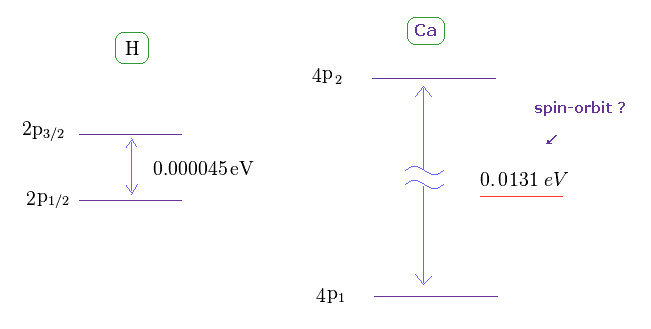The triplet energy intervals of Ca is much larger than hydrogen 2p doublet.
As I said above, spin-spin interaction has NO relation with this triplet.
So there is ONLY spin-orbit coupling left, if "spin" is involved in this triplet.

(Fig.36) Effective core charge Z becomes unrealistically too large. ( Z = 2 → 6.946. )Like in this section, we suppose triplet of Ca is due to spin-orbit coupling, and calculate effective core charge Z.
If so, this charge Z becomes unrealistically too big ( Z = 6.946 ) to explain large energy interval of 0.0131 eV.
As a result, spin-orbit coupling proves to be NOT involved in this triplet, either.

(Fig.37) True effective charge Z = 2.68.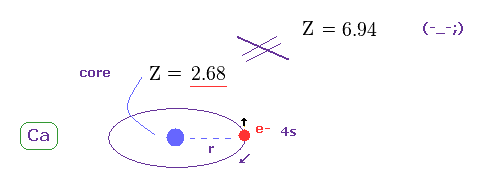Using the first ionization energy of 6.113 eV, we can know the true effective core charge Z, like in this section.
This true effective charge (= 2.68 ) based on ionization energy is much smaller than Z = 6.94.

Again, spin-orbit coupling proves to have NO relation with triplet splitting.

## Strontium (= Sr ) triplet does NOT represent "Spin", either.

(Fig.38) Triplet, Singlet splitting in Sr is too large for spin-spin (-orbit) coupling.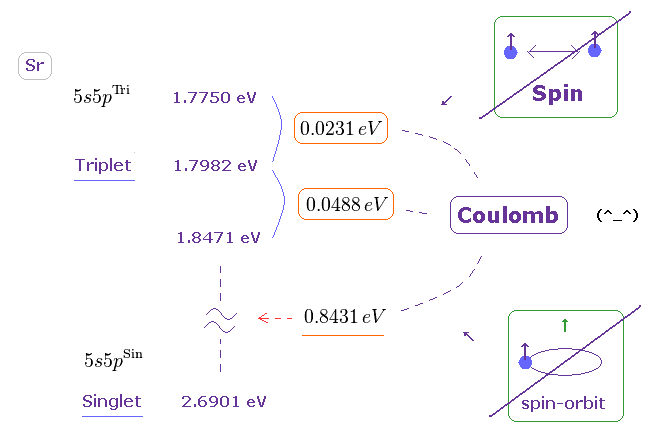Triplet and singlet energy levels are shown on this site.
Converting energy unit ( cm-1 → eV ) byWe obtain Fig.38.
If you compare Fig.38 (= Sr ) with Fig.30 (= Mg ), you will understand energy splitting (in triplet ) in Sr is much larger than Mg.
So it is much more impossible that spin-spin ( or -orbit ) coupling is involved in this triplet.

(Fig.39) Radius of helium-like model in Sr.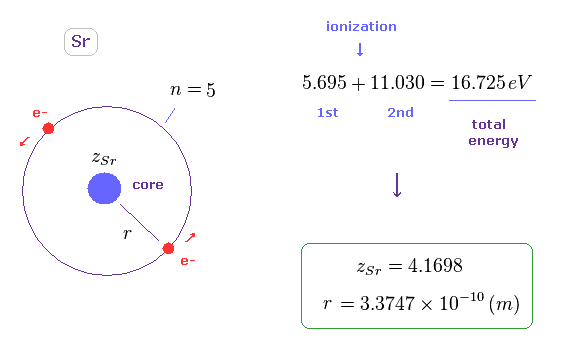The sum of 1st and 2nd ionization energy is 16.725 eV in Sr, as shown on this site.
Like Fig.15, we suppose helium-like model in Sr, and its total energy is -16.725 eV.

In this model, the radius (= r ) becomes 3.3747 Å, which is longer than Mg.
The calculation method is the same as this section.

(Fig.40) Spin-spin magnetic energy is 1/10000 times triplet splitting in Sr.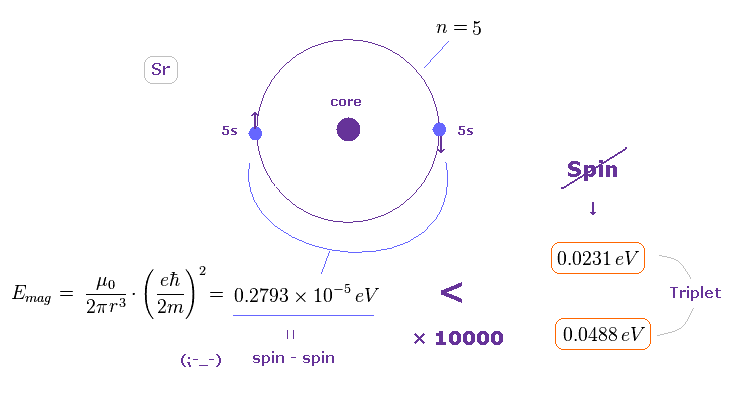Using the approximate radius of Fig.39, we find the spin-spin magnetic dipole energy in Sr outer orbital becomes very small.
(= 0.2793 × 10-5 eV, about the method, see this section. )
This result shows it is impossible to explain triplet splitting by spin-spin magnetic interactions also in Sr.

### [ How about spin-orbit coupling energy in Sr ? ]

(Fig.41) Doublet of H 2p and triplet of Sr 5p.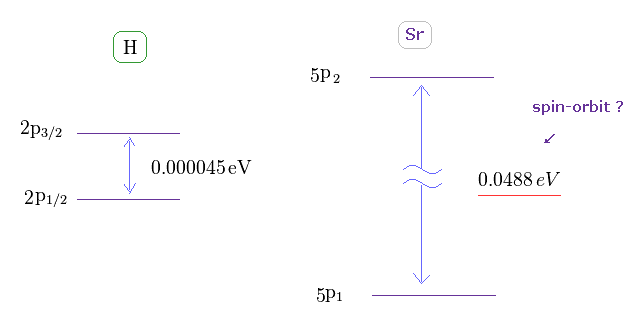The triplet energy intervals of Sr is much larger than hydrogen 2p doublet.
As I said above, spin-spin interaction has NO relation with this triplet.
So there is ONLY spin-orbit coupling left, if "spin" is involved in this triplet.

(Fig.42) Effective core charge Z becomes unrealistically too large. ( Z = 2 → 11.409 )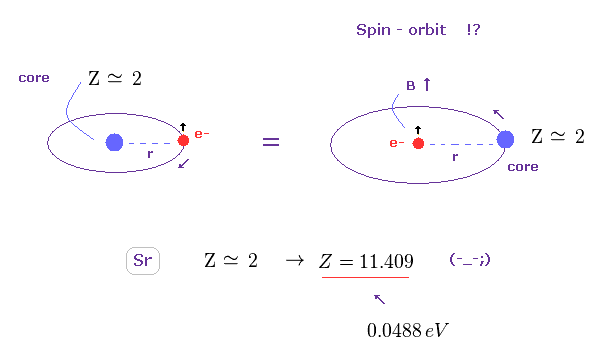Like in this section, we suppose triplet of Sr is due to spin-orbit coupling, and calculate effective core charge Z.
If so, this charge Z becomes unrealistically too big ( Z = 11.409 ) to explain large energy interval of 0.0488 eV.
As a result, spin-orbit coupling proves to be NOT involved in this triplet, either.

(Fig.43) True effective charge Z = 3.23.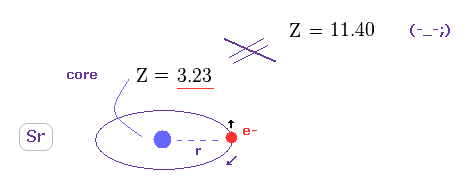Using the first ionization energy of 5.695 eV, we can know the true effective core charge Z, like in this section.
This true effective charge (= 3.23 ) based on ionization energy is much smaller than Z = 11.409.

Again, spin-orbit coupling proves to have NO relation with triplet splitting.

## Barium (= Ba ) triplet does NOT represent "Spin", either.

(Fig.44) Triplet, Singlet splitting in Ba is too large for spin-spin (-orbit) coupling.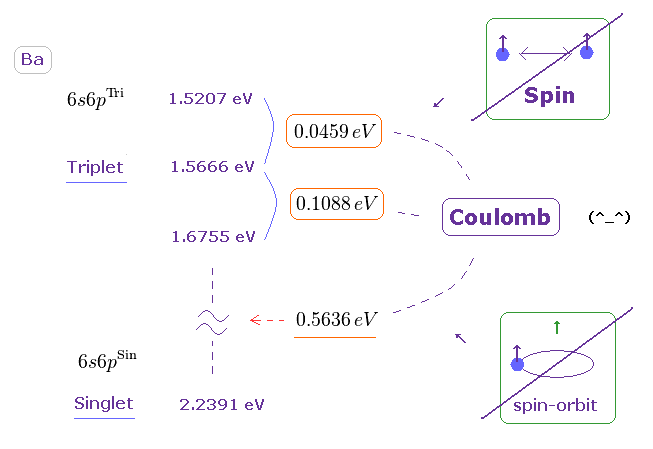Triplet and singlet energy levels are shown on this site.
Converting energy unit ( cm-1 → eV ) byWe obtain Fig.44.
If you compare Fig.44 (= Ba ) with Fig.30 (= Mg ), you will understand energy splitting (in triplet ) in Ba is much larger than Mg.
So it is much more impossible that spin-spin ( or -orbit ) coupling is involved in this triplet.

(Fig.45) Radius of helium-like model in Ba.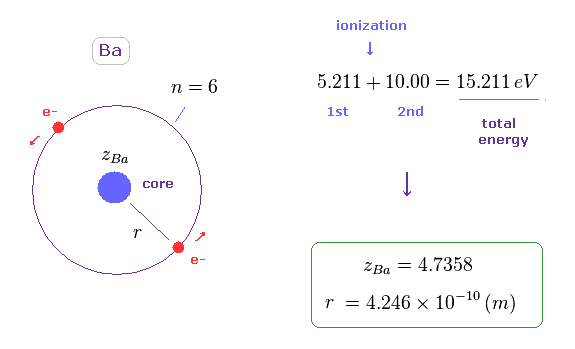The sum of 1st and 2nd ionization energy is 15.211 eV in Ba, as shown on this site.
Like Fig.15, we suppose helium-like model in Sr, and its total energy is -15.211 eV.

In this model, the radius (= r ) becomes 4.246 Å, which is longer than Mg.
The calculation method is the same as this section.

(Fig.46) Spin-spin magnetic energy is 1/10000 times triplet splitting in Ba.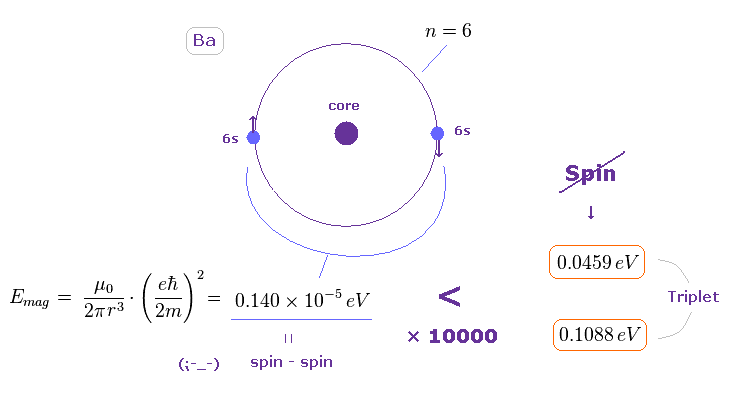Using the approximate radius of Fig.45, we find the spin-spin magnetic dipole energy in Ba outer orbital becomes very small.
(= 0.140 × 10-5 eV, about the method, see this section. )
This result shows it is impossible to explain triplet splitting by spin-spin magnetic interactions also in Ba.

### [ How about spin-orbit coupling energy in Ba ? ]

(Fig.47) Doublet of H 2p and triplet of Ba 6p.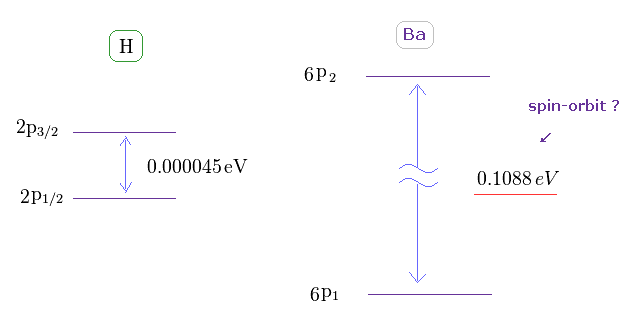The triplet energy intervals of Ba is much larger than hydrogen 2p doublet.
As I said above, spin-spin interaction has NO relation with this triplet.
So there is ONLY spin-orbit coupling left, if "spin" is involved in this triplet.

(Fig.48) Effective core charge Z becomes unrealistically too large. ( Z = 2 → 15.984 )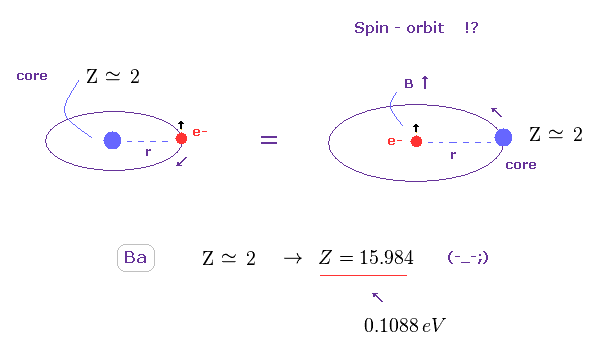Like in this section, we suppose triplet of Ba is due to spin-orbit coupling, and calculate effective core charge Z.
If so, this charge Z becomes unrealistically too big ( Z = 15.984 ) to explain large energy interval of 0.1088 eV.
As a result, spin-orbit coupling proves to be NOT involved in this triplet, either.

(Fig.49) True effective charge Z = 3.71.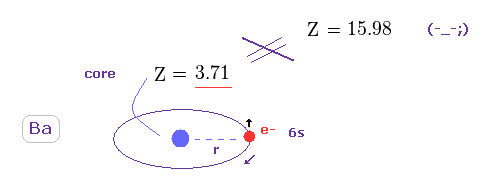Using the first ionization energy of 5.211 eV, we can know the true effective core charge Z, like in this section.
This true effective charge (= 3.71 ) based on ionization energy is much smaller than Z = 15.984.

Again, spin-orbit coupling proves to have NO relation with triplet spilitting in Ba.

## Quantum numbers in triplet and singlet are strange.

(Fig.50) Energy levels of Calcium (= Ca ).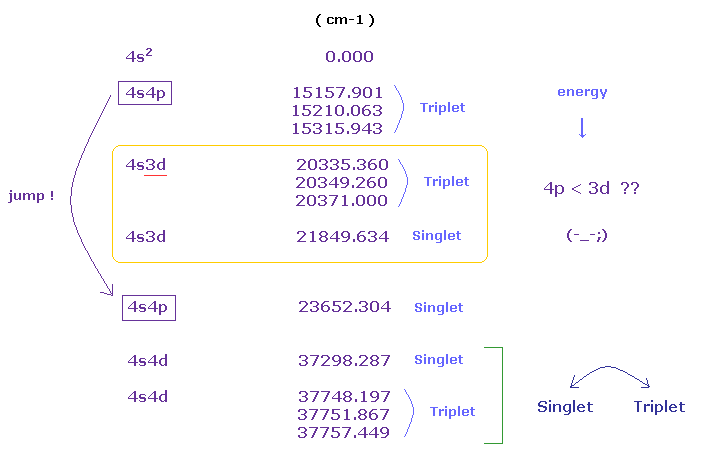If you see the energy levels of Ca (= Nist ) carefully, you will find quantum numbers and their orders are very unreasonable.
In usual periodic table, "3d" orbitals are lower than 4p orbitals in the energy levels.

But as you see Fig.50, 4p orbital (= triplet ) is lower than 3d orbitals.
( In Fig.50, the top (= 4s2 ) is ground state, and the lower configuration means higher energy levels. )

And in 4s4d configuration, the order of triplet and singlet is reversed.
( Usually, triplet → singlet. But in 4s4d, singlet → triplet. )

## Magnitude of energies and quantum numbers are "chaotic".

(Fig.51) Energy levels of Mg and Sr.   "4f" appears ONLY in Mg ?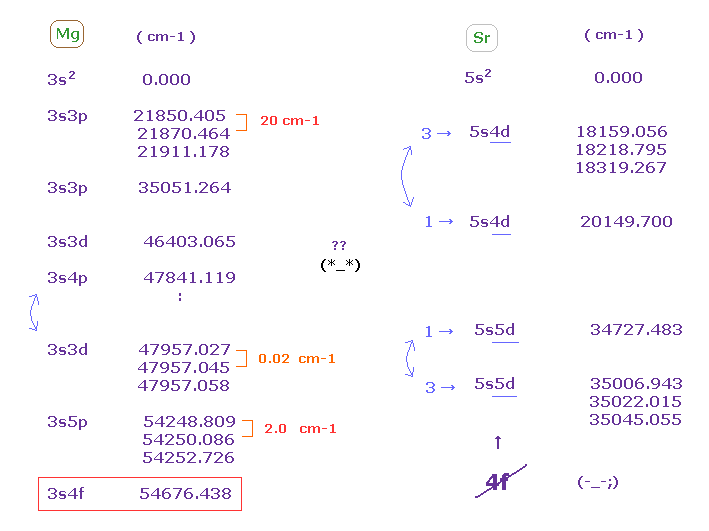In magnesium, the energy intervals of triplets are chaotic.
( 3s3p-- 20 cm-1,   3s3d-- 0.02 cm-1,   3s5p-- 2.0 cm-1 ).
And "4f" energy levels exist only in magnesium, but don't exist in strontium and barium .
This is strange.

Though "3d" orbital is lower than "4p" in energy levels, but in Mg, "3s4p" becomes lower than "3s3d".
This is strange, too.
In Sr, "triplet" (= 3 ) appears first in 5s4d, but "singlet" (= 1 ) appears first in 5s5d.
Again, this is one of artificial manipulations.

So the concepts of these triplet and singlet were produced by considering ONLY unrealistic quantum numbers.
They completely ignored the magnitude of energy ( ← though very important ! ) and their orders.

## Supplement.

### [ Magnetic moment in elliptical orbits. ]

(Sp.1)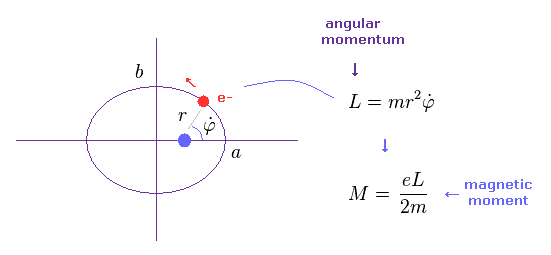In the upper section, we deal with simple circular orbit.
In this section, we show the magnetic moment of elliptical orbit becomes the same as circular orbit like Sp.1

(Sp.2)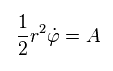In the system of central force, the areal velocity (= A ) is constant like Sp.2.

(Sp.3)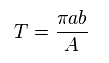The total area of ellipse is equal to "πab".
Time period (= T ) of one-orbit is given by the total area (= πab ) / A.
Using Sp.2 and Sp.3, the current I becomes

(Sp.4)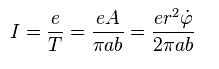The magnetic moment (= M ) is given by the current I × area.
( When you divide some area into infinitesimal loops, you will find this law holds in any forms of current. )
As a result,

(Sp.5)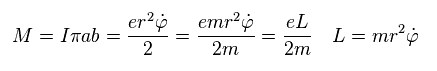"3p" orbital has the angular momentum of 1 × ħ.
Substituting ħ into Sp.5, we obtain the Bohr magneton as the orbital magnetic moment of "3p".

(Sp.6) 3p = Bohr magneton.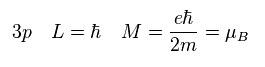### [ Calculation of average radius of magnesium 3s orbital ]

(Sp.7) 3s outer orbitals of Magnesium.In Sp.7 magnesium model, two 3s outer electrons are moving on the common orbit at the velocity "v", on the opposite sides of the nucleus.
The effective positive charge of the core (= Mg nucleus + all inner electrons ) is "z".
And the orbital radius is "r".

Equating the centrifugal force to the Coulomb force, we have
(Sp.8)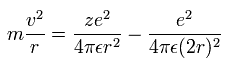where "m" is the electron mass.
The left side is centrifugal force, and the first term of the right side is Coulomb attractive force between the core and each electron.
The second term is the repulsive force between two electrons.

The circular orbital length is supposed to be an integer (n) times de Broglie wavelength ( λ = h/mv ) of the electron.
(Sp.9)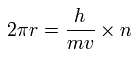The total energy E is the sum of the kinetic and the Coulomb potential energy of the two electrons, so
(Sp.10)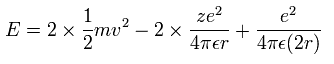Suppose the total energy E of Sp.10 is equal to the sum of 1st and 2nd ionization energies ( E = -22.681 eV ).
Solving the the simultaneous equations of Sp.8-Sp.10, we can obtain the radius "r" and effective charge "z".
From Sp.9,

(Sp.11)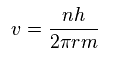Substituting Sp.11 into Sp.8, we have

(Sp.12)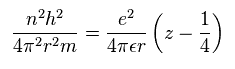From Sp.12

(Sp.13)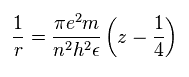Substituting Sp.11 and Sp.13 into Sp.10,

(Sp.14)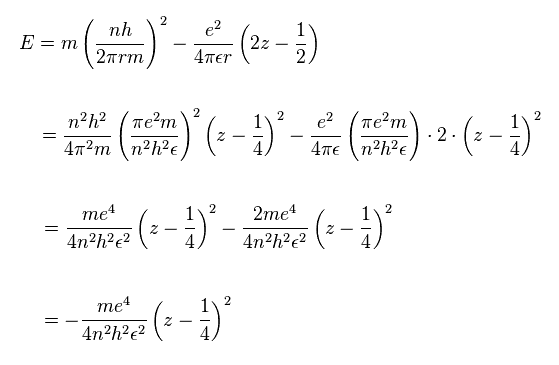Here we treat Mg with two valence electrons as helium-like atom.
The total energy of these two outer electrons is supposed to be the sum of 1st and 2nd ionization energies ( see this site ) like Sp.15.

(Sp.15)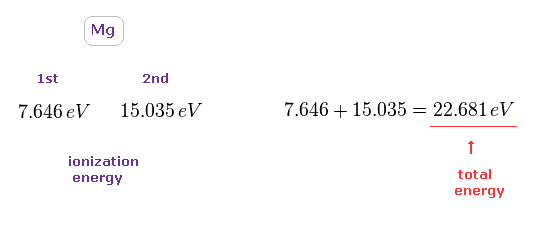Substituting Sp.15 ( "eV" → "J" ) and n = 3 into Sp.14,

(Sp.16)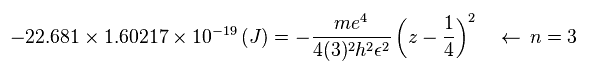Using the following values,

(Sp.17)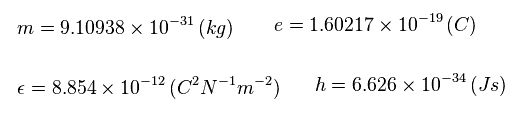The central effective charge z becomes

(Sp.18)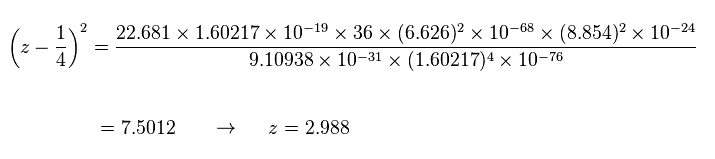Substituting z = 2.988 and n = 3 into Sp.13, the radius "r" becomes

(Sp.19)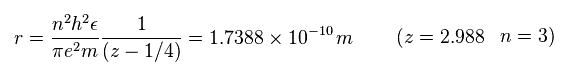2014/2/21 updated. Feel free to link to this site.